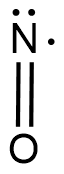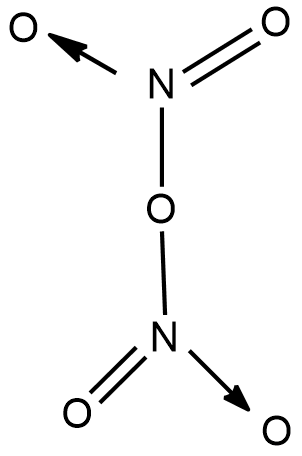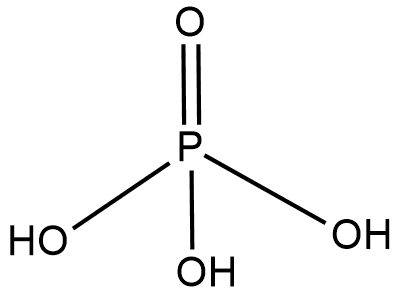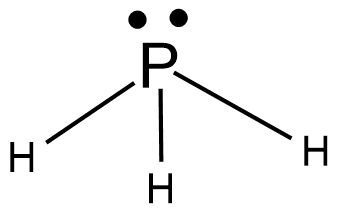Courses
Courses for Kids
Free study material
Offline Centres
MoreLast updated date: 07th Dec 2023
Total views: 279.9k
Views today: 5.79k

# Write the molecular formula and the structural formula for the following molecules.(A) Nitric acid (B) Dinitrogen pentoxide(C) Phosphoric acid(D) PhosphineVerified
279.9k+ views
Hint : The molecular formula of a compound is defined as the number of moles or atoms of one element reacting with another element having them in the lower cases and instead of the full name, we have the element's short forms used in the periodic table. Now the structural formula is the representation of the structure of the compound after it forms the compound.

In the question we are asked to give the molecular formula of the following compound and their structural formula, first let’s define the two terms.
Molecular formula is defined as the total number of atoms of each element in each molecule of the compound. The total number of atoms of each element being reacted depends upon the number of electrons the element loses or gains.
Structural formula is defined as the structure showing the bonds between these elements with proper geometry in a three-dimensional space.
- Nitric acid
Now in this we know that the nitrogen forms an acid and that too with oxygen because it is one of the few higher electronegative elements. The term nitric says that the nitrogen doesn’t lose any electrons as such and forms two covalent bonds so as to satisfy oxygen’s valency but its valency is not satisfied.
Molecular formula - $NO$- Dinitrogen peroxide
As the name already suggests that it is a compound formed by nitrogen and oxygen and two atoms of nitrogen are present with oxygen and the oxygen atom exists between the nitrogen atom and attached to the nitrogen atom so as to complete their octane.
Molecular formula- ${N_2}{O_5}$- Phosphoric acid
Phosphoric acid is an acid formed by phosphorus. Phosphorus belongs to the nitrogen family and has five electrons that are not that tightly bound therefore the phosphorus shares all the electrons with oxygen and hydroxide ions.
Molecular formula- ${H_3}P{O_4}$Phosphine
Phosphine is a gas emitted when phosphorus is reacted in an aqueous solution with potassium carbonate. The phosphorus gets reacted heavily with hydrogen to form a gas named phosphine. Its three electrons get bonded while one pair remains unreacted.
Molecular formula- $P{H_3}$Note :
The presence of lone pairs very much affects the geometry of the compound and also how the structure of the compound would be formed. The lone pair also provides hindrance and repulsion to the bonded electrons. So, the knowledge of the bonded and unbonded electrons is very important.